# How to get a week start and end date based on a specific date in Excel?

In this article, the user will learn how to obtain week start and end date based on a specific date in Excel. The two examples are depicted in this article. Both examples are based on the starting and end date of the provided week. The first example uses a formula to obtain the start and end date for the week. This example briefs the use of weekday and weekend methods to perform the same task. On the other hand, the second example will allow users to understand the use of VBA code. VBA code example also allows users to use the predefined method to perform the same task. Both the specified methods are easy and efficient to process data.

## Example 1: To get the week start and end date based on the specific date in excel by using the formula

### Step 1

This example allows the user to understand the formula to obtain the star and end date of the week. Consider the below given sample worksheet. This worksheet contains a date column and the week start date and weekend date column to store the processed data.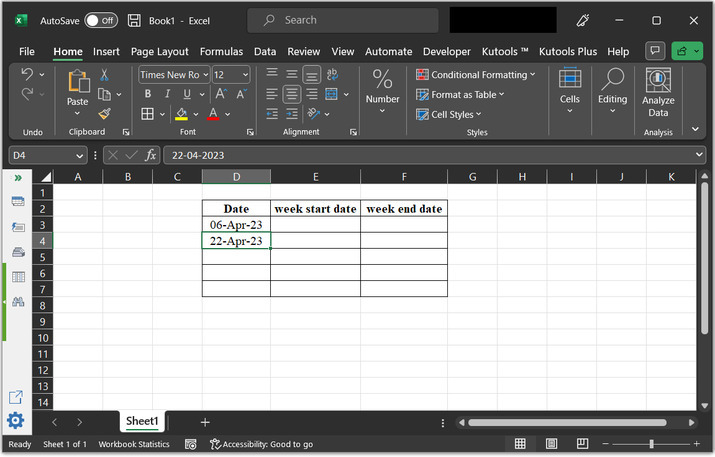### Step 2

After that let’s process the data to first obtain the week start date. After that type “=D3-weekday(D3,2)+1” to the cell, and press “enter key”.

### Explanation of the above formula

D3 cell contains date values. Weekday function is used to get the day of the week for a given date, with 0 representing Sunday and 6 representing Saturday. The second argument of the D3-weekday function is an optional parameter that specifies the day of the week considered as the first day of the week. In this case, the second argument is 2, which means that the week starts on Tuesday.

The plus “1” at the end of the expression is simply adding 1 to the result of the D3-weekday function. This is often used to shift the day of the week representation by one day. In this case, it would shift the representation so that 1 represents Tuesday, 2 represents Wednesday, and so on, up to 7 represents Monday.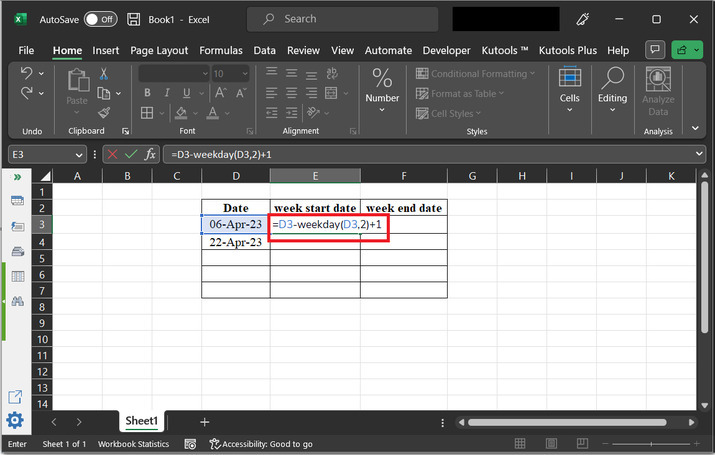### Step 3

Press the “Enter” key to the above formula. This will display the results as given below −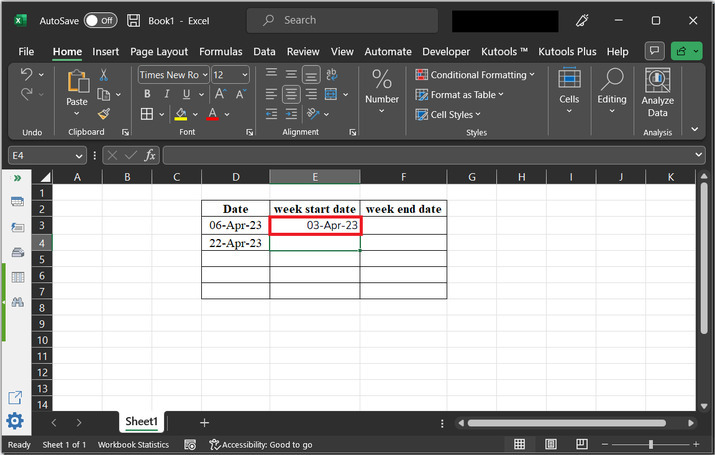### Step 4

Now, let’s evaluate the weekend date. After that type “=D3+7-WEEKDAY(D3,2)” to the cell

### Explanation

Breaking down the formula, "=D3+7", and this will add seven days to the date in cell D3.

The second part is "WEEKDAY(D3,2)". This calculate the weekday of the date in cell D3, where weekday 1 represents Sunday and weekday 7 represents Saturday. The second argument, "2", tells Excel to use a different numbering system for the weekdays, where weekday 1 represents Monday and weekday 7 represents Sunday.

Subtracting the weekday from the date added with 7 days ensures that the resulting date falls on a Monday. This formula is often used to generate a series of dates that fall on Mondays, such as for weekly reports or schedules.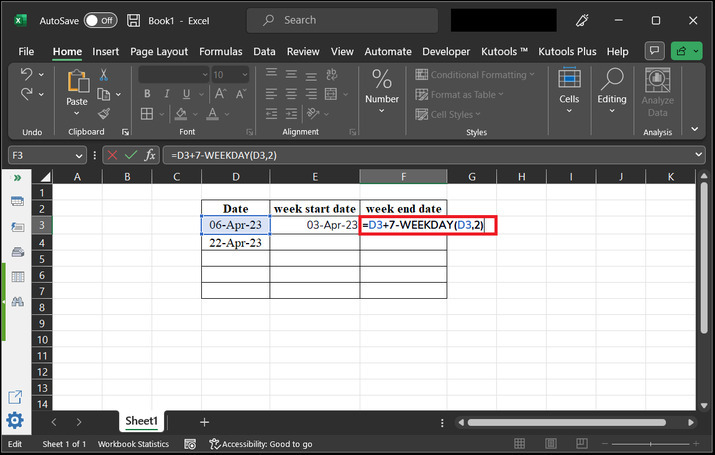### Step 5

Press “Enter” key. This will display the date as shown below −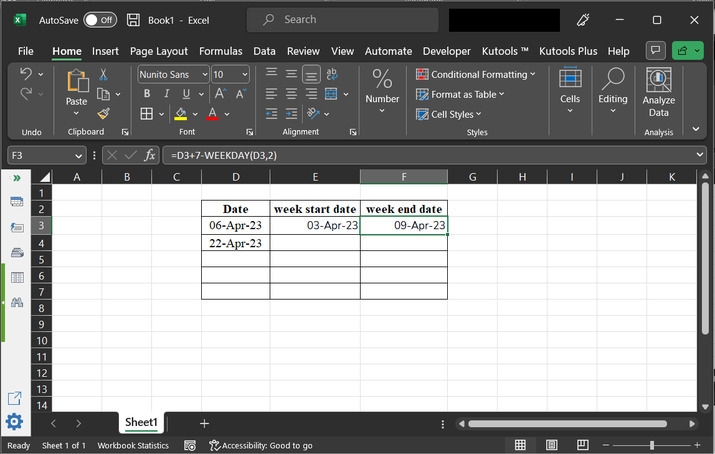### Step 6

After that, to copy the formula of rows go to the E3 cell and drag the plus “+” sign to the bottom of the rows. Consider depicted image −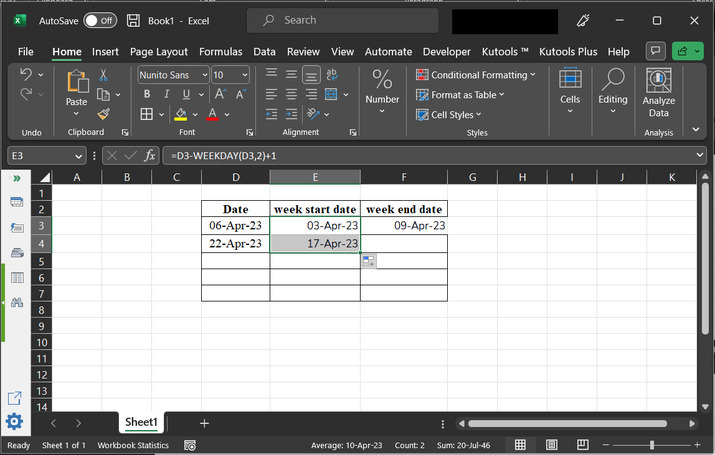### Step 7

After that go to the F column, and perform the same task, as given below. Consider the below-given image for reference −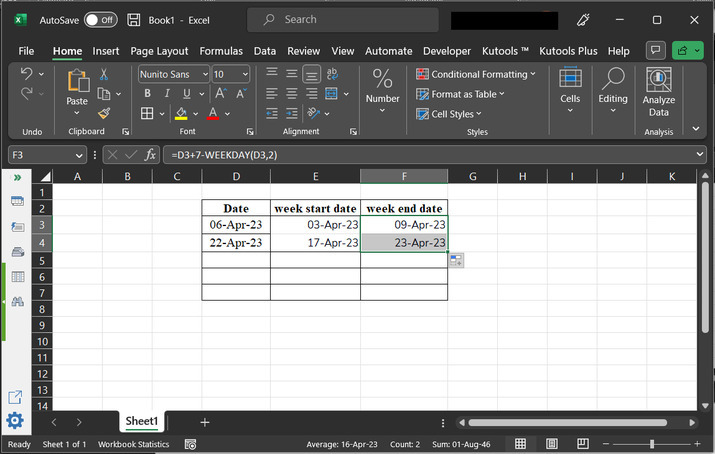## Example 2: To get the week start and end date based on the specific date in excel by using the VBA code

### Step 1

To use VBA code to perform the same task. Consider the given worksheet below. Go to the “Developer” tab and then select the option “Visual Basic” under the code section.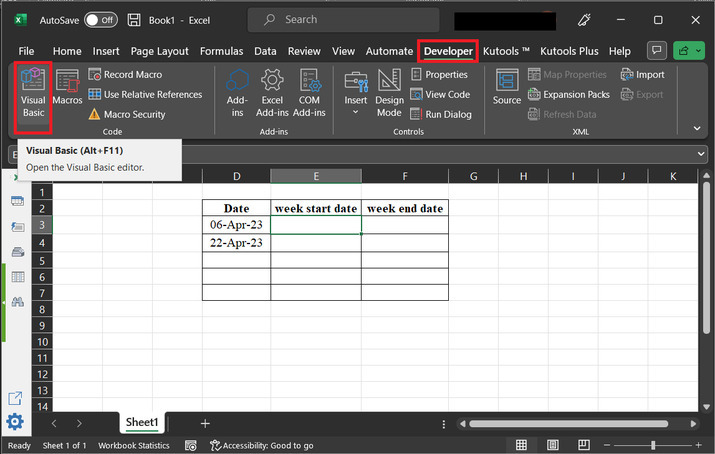### Step 2

This will open the “Microsoft Visual for Basic Applications”, as depicted below −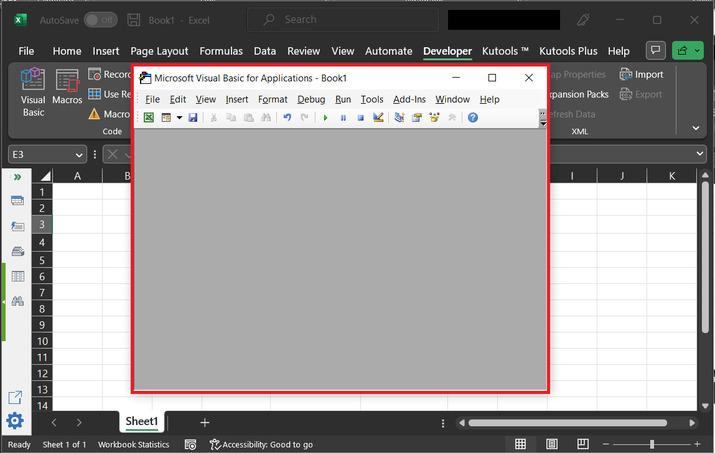### Step 3

In the dialog box, click on the “Insert” option, then select the option “Module” option.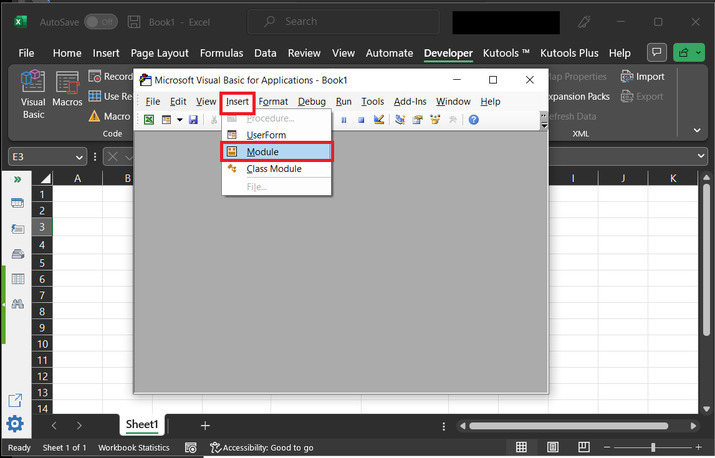### Step 4

Consider the below-given code −

' function definition header
Function GetWeekStartDate(inputDate As Date) As Date
'Calculate the week start date
GetWeekStartDate = inputDate - Weekday(inputDate, vbMonday) + 1
' function end
End Function


Consider code snapshot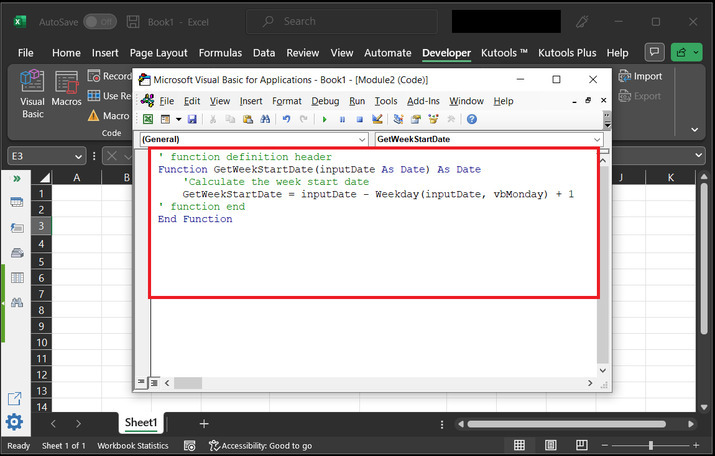### Step 5

Again, open the E3 cell, and generate a function call “=GetWeekStartDate(D3)”.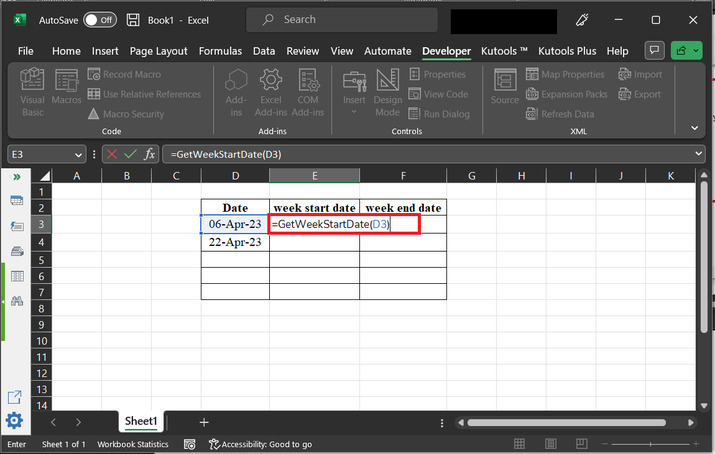### Step 6

Press “Enter” key. This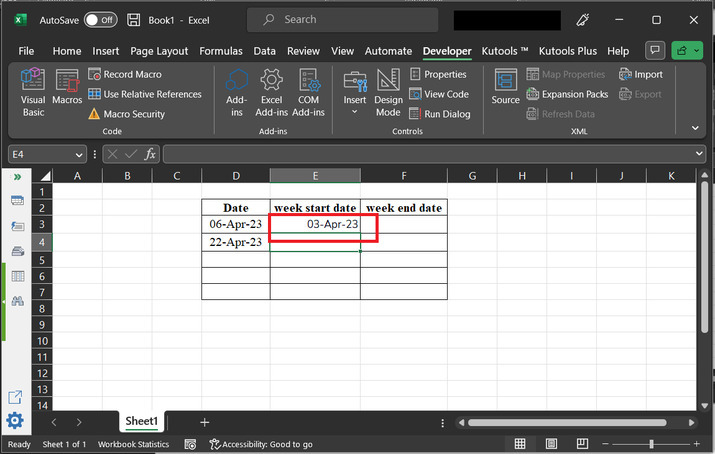### Step 7

Now, to evaluate the weekend date. Go to the F3 cell.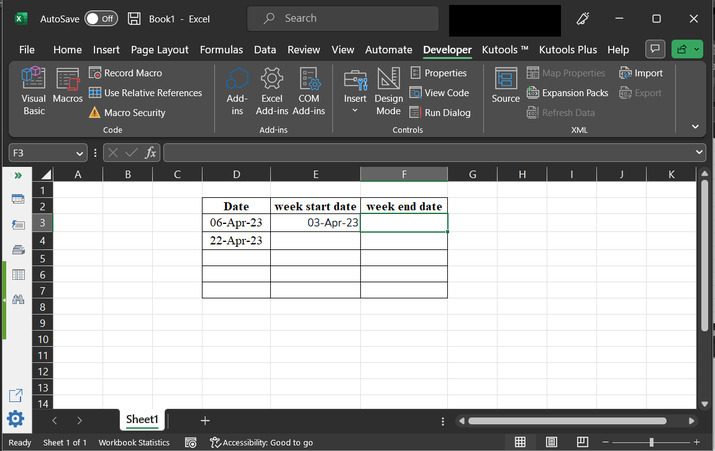### Step 8

Again open the visual basic editor to create another method. Go to the “Developer” tab and then select “Insert” and then “Visual Basic” under the code section. As, depicted below −### Step 9

After that select the “Insert” tab and then choose “Module”.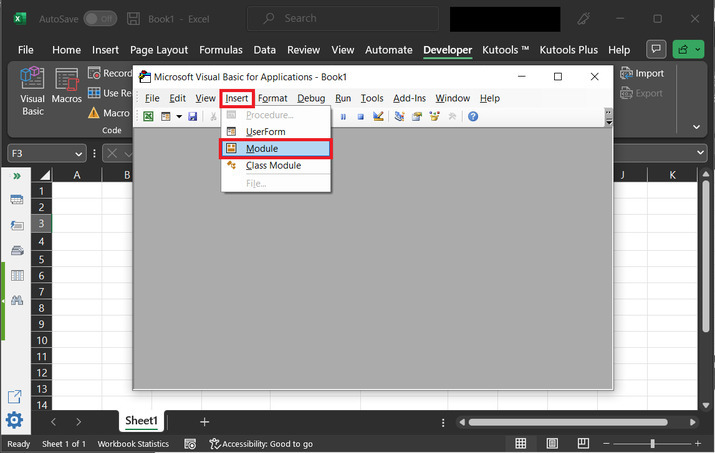### Step 10

Type the below-given code into the code area −

' define function header
Function GetWeekEndDate(inputDate As Date) As Date
'Calculate the week end date
GetWeekEndDate = inputDate + (7 - Weekday(inputDate, vbMonday))
End Function


Code snapshot −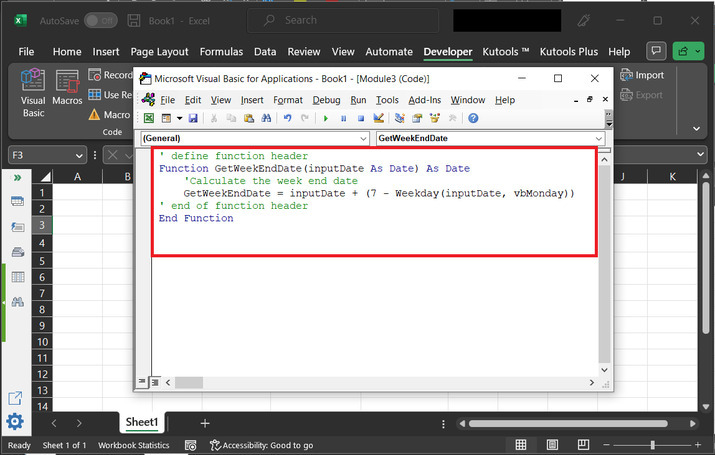### Step 11

After that Go to the F3 cell “=GetWeekEndDate(D3)”. This will generate a function call to above-declared method.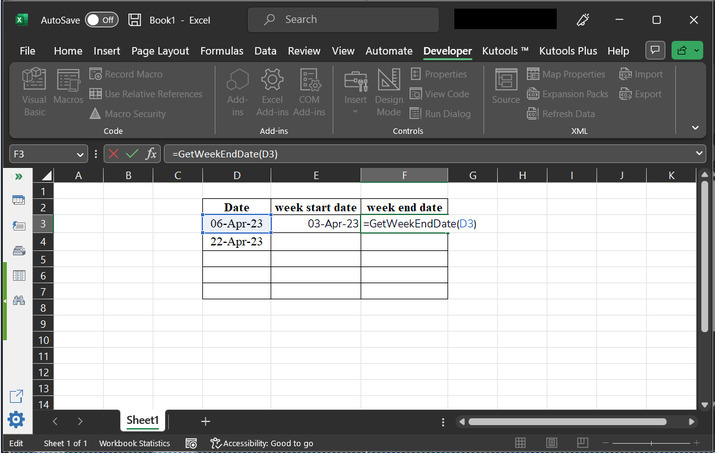### Step 12

Press the “Enter” key, and this will display the below-given results on the sheet.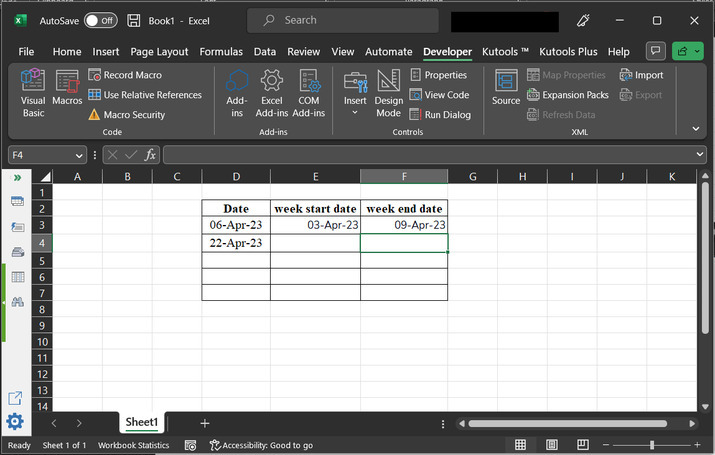### Step 13

To copy the formula, of the E3 cell to the E4 cell simply click on the bottom of the E3 cell, and drag the plus sign to the bottom of the cell.### Step 14

Perform same task with the F column.## Conclusion

After the successful completion of this article, the user will able to understand the process to get the week start and end date from a provided date. This article guides the user about the way so, that the user can easily perform the same task with any of the specified techniques, that is either by using a formula or by using the VBA code.

Updated on: 08-May-2023

726 Views